## How to count / sum odd / even numbers or rows in Excel?

If there are range of numbers in your worksheet which contain both odd numbers and even numbers, but now, you need to count or sum only the odd numbers or even numbers. How could you finish this task quickly in Excel worksheet?

Count odd or even numbers with formulas in Excel

Sum odd or even numbers with formulas in Excel

Sum odd or even rows with formulas in Excel

#### Count odd or even numbers with formulas in Excel

To count only the even or odd numbers in a range, the following formulas can help you, please do as these:

1. In order to count the odd numbers only, enter this formula =SUMPRODUCT((MOD(A1:C8,2)<>0)+0) in a blank cell,, see screenshot: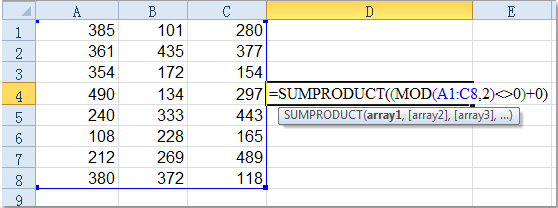2. Then press Enter key, and you will get the number of odd values in the selected range.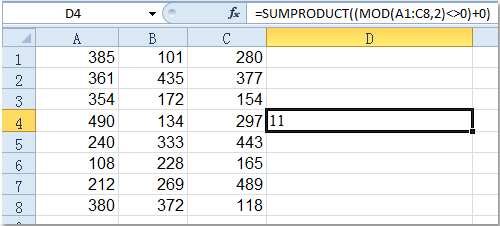Notes:

1. If you want to get the number of even values only, please apply this formula =SUMPRODUCT((MOD(A1:C8,2)=0)+0).

2. In above formulas, A1:C8 is the range that you want to count the odd or even numbers, you can change it as you need.

#### Sum odd or even numbers with formulas in Excel

If you need to add up all the odd or even numbers only in a range, you can use the following formulas: normal formulas or array formulas.

1. Normal formulas for summing odd/even numbers in a range:

Please enter this formula =SUMPRODUCT(--(MOD(A1:C8,2)=1),A1:C8) into a blank cell, see screenshot: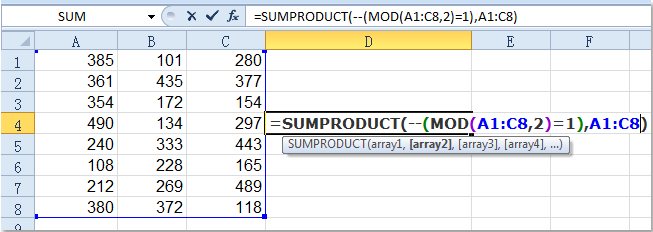And then press Enter key, all the odd values have been added up in the range.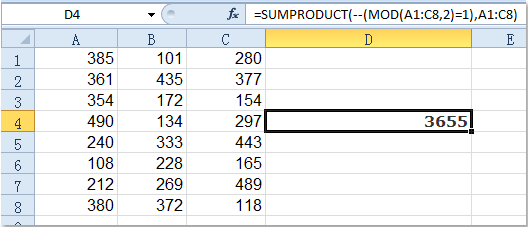Notes：1.To sum only the even numbers, you can use this formula: =SUMPRODUCT(--(MOD(A1:C8,2)=0),A1:C8).

2. In the above formulas, A1: C8 is the range you want to add up the odd / even numbers in.

2. Array formulas for summing odd/even numbers in a range

The following array formulas also can help you to deal with this problem.

In a blank cell, please type this formula =SUM(IF(MOD(\$A\$1:\$C\$8,2)=1,\$A\$1:\$C\$8)), then press Ctrl+Shift+Enter keys together. And you will get the total of the odd numbers.

For summing the even numbers, please apply this array formula =SUM(IF(MOD(\$A\$1:\$C\$8,2)=0,\$A\$1:\$C\$8)), and remember to press Ctrl+Shift+Enter keys.

####Sum odd or even rows with formulas in Excel

Sometimes, you need to sum a range of cells that locate in only even-numbered or odd-numbered rows. You can use formulas to solve it as well.

1. Supposing you have the following data, and now to sum odd rows in Column B, please input this formula =SUMPRODUCT(B2:B10,MOD(ROW(B2:B10)+0,2)) into a blank cell. See screenshot: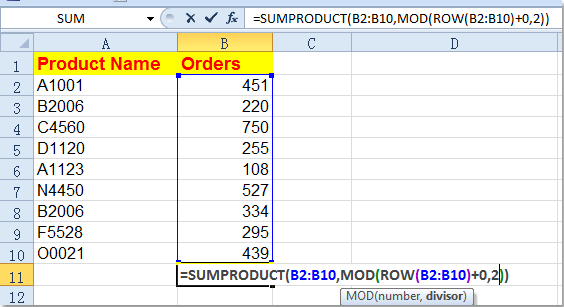2. Then type Enter key, and all the values of odd rows have been added up.Notes:

1. To sum the even –numbered rows, please use this formula =SUMPRODUCT(B2:B10,MOD(ROW(B2:B10)+1,2)).

2. In the above formulas, B2:B10 is the range that you want to use, you can modify it as your need.

#### Count/average/sum odd or even rows with Kutools for Excel

If you have Kutools for Excel installed, you can quickly select the odd or even rows or column, then view the calculations in status bar.

 with more than 300 handy functions, makes your jobs more easier. Free Downloadfree full-featured in 30 days

After Kutools for Excel, please do as below:

1. Select the whole range of rows, and click Kutools > Select > Select Interval Rows & Columns. See screenshot: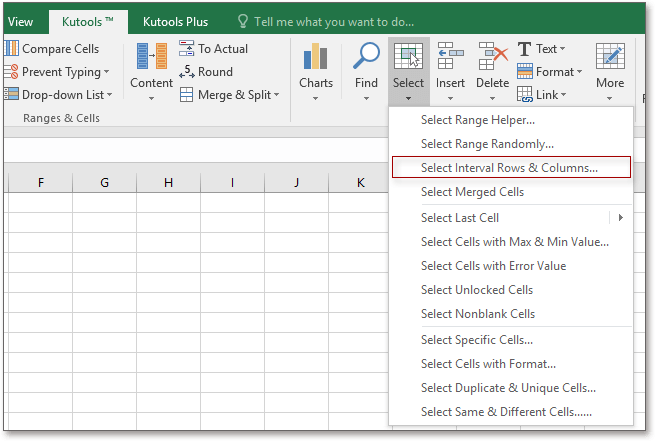2. Then in the popping dialog, check Rows or Columns as you need, and type 1 into both of textbox, uncheck Select entire rows. See screenshot: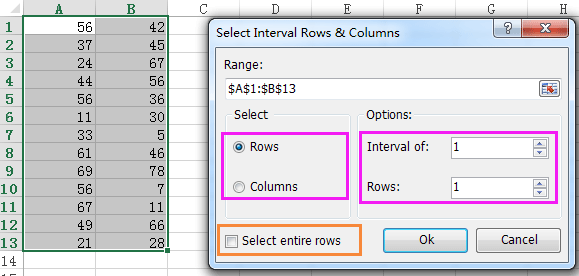3. Click Ok, now all the odd rows are selected, and go to status bar, you can view the average, counting, and summing results.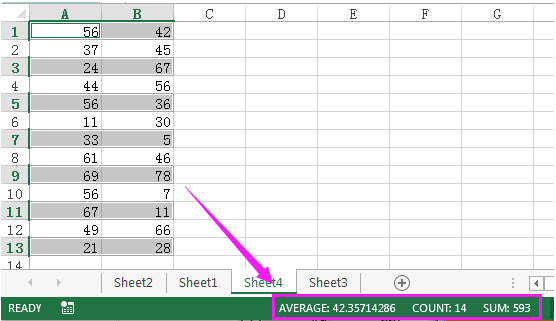Tip: If you want to count, average or sum even rows, just select all rows except first row, then apply Select Interval Rows & Columns.

Related article:

How to highlight odd or even numbers in Excel?

### Best Office Productivity Tools

Supercharge Your Spreadsheets： Experience Efficiency Like Never Before with Kutools for Excel

 Popular Features: Find/Highlight/Identify Duplicates   |  Delete Blank Rows   |  Combine Columns or Cells without Losing Data   |   Round without Formula ... Super Lookup: Multiple Criteria VLookup  |   Multiple Value VLookup  |   VLookup Across Multiple Sheets   |   Fuzzy Lookup .... Advanced Drop-down List: Quickly Create Drop Down List   |  Dependent Drop Down List   |  Multi-select Drop Down List .... Column Manager: Add a Specific Number of Columns   |   Move Columns   |   Unhide Columns   |   Compare Columns to Select Same & Different Cells ... Featured Features: Grid Focus   |  Design View   |   Big Formula Bar   |  Workbook & Sheet Manager   |  Resource Library (Auto Text)   |  Date Picker   |  Combine Worksheets   |  Encrypt/Decrypt Cells   |  Send Emails by List   |  Super Filter   |   Special Filter (filter bold/italic/strikethrough...) ... Top 15 Toolset:  12 Text Tools (Add Text, Remove Characters, ...)   |   50+ Chart Types (Gantt Chart, ...)   |   40+ Practical Formulas (Calculate age based on birthday, ...)   |   19 Insertion Tools (Insert QR Code, Insert Picture from Path, ...)   |   12 Conversion Tools (Numbers to Words, Currency Conversion, ...)   |   7 Merge & Split Tools (Advanced Combine Rows, Split Cells, ...)   |   Many More...

Kutools for Excel boasts over 300 features, ensuring that what you need is just a click away...

Supports Office/Excel 2007-2021 & newer, including 365   |   Available in 44 languages   |   Enjoy a full-featured 30-day free trial.#### Office Tab Brings Tabbed interface to Office, and Make Your Work Much Easier

• Enable tabbed editing and reading in Word, Excel, PowerPoint, Publisher, Access, Visio and Project.
• Open and create multiple documents in new tabs of the same window, rather than in new windows.
• Increases your productivity by 50%, and reduces hundreds of mouse clicks for you every day!